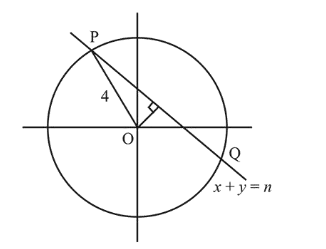# The sum of the squares of the lengths

Question:

The sum of the squares of the lengths of the chords intercepted on the circle, $x^{2}+y^{2}=16$, by the lines, $x+y=\mathrm{n}$, $n \in N$, where $N$ is the set of all natural numbers, is :

1. (1) 320

2. (2) 105

3. (3) 160

4. (4) 210

Correct Option: , 4

Solution:

Let the chord $x+y=n$ cuts the circle $x^{2}+y^{2}=16$ at $P$ and $Q$ Length of perpendicular from $\mathrm{O}$ on $P Q$

$=\left|\frac{0+0-n}{\sqrt{1^{2}+1^{2}}}\right|=\frac{n}{\sqrt{2}}$Then, length of chord $P Q=2 \sqrt{4^{2}-\left(\frac{n}{\sqrt{2}}\right)^{2}}$

$=2 \sqrt{16-\frac{n^{2}}{2}}$

Thus only possible values of $n$ are $1,2,3,4,5$.

Hence, the sum of squares of lengths of chords

$=\sum_{n=1}^{5} 4\left(16-\frac{n^{2}}{2}\right)$

$=64 \times 5-2 \cdot \frac{5 \times 6 \times 11}{6}=210$